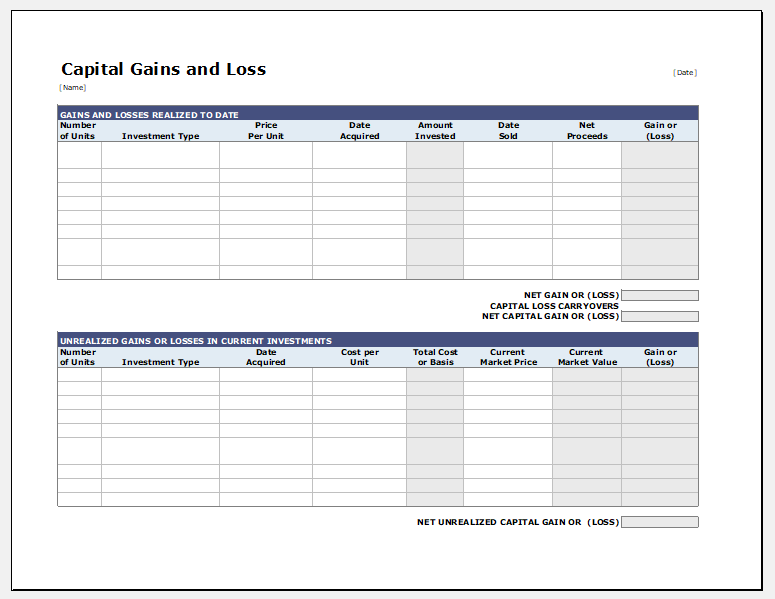# Capital Gains and Losses Calculator

Paying the tax is an obligation. When it comes to calculating the tax, you are required to pay, you should have a look at all your income and sources of income. The income generated by selling the capital assets as well as stocks and property should also be included in the income on which tax is to be paid.

It is not advisable to pay the taxes on all the sales prices. The best thing to do is to calculate capital gains and losses. It is recommended to make a report about your capital gains and losses so that you can form a file for returns.

## Capital gains and losses:

The capital gains are generally obtained from selling the assets owned by a person. The capital gains from the assets which you own for less than a year are known as short-term capital gains. Whereas, the capital gains from selling the assets which you own for more than a year are known as long-term capital gains. The long-term capital gains have a lower tax rate.

In order to know whether you have gotten capital gain or suffered from capital loss, you are required to keep track of everything you sold. For this, you should also consider how much you have paid in order to acquire the capital.

### How to calculate capital gains and losses?

As a matter of fact, the tax is required to be paid on every capital gain. How much tax you are needed to pay depends on capital gain and losses? So, calculating the capital gains and losses before paying the taxes is inevitable in this situation.

There are a number of online tools and calculators that are helpful in determining the value of capital gains and losses. It is very simple to calculate the capital losses and gains by uses any available online tool. The tool to be used should be efficient enough to give accurate results.

The simple way is to calculate the capital gains and losses is to subtract the basis from the amount you get after completely paying the expenses of the sales. Similarly, if you want to calculate the percentage capital gains or losses, you are required to divide the gain or loss by basis and then multiply it by 100. The result obtained from the calculation is the profit or loss in the perform of percentage.

### Capital gains and losses calculator template:

Calculator template worksheet prepared in MS Excel is a useful tool that helps in calculating gains and losses. Moreover, this template also helps a user in organizing the data so that it can help for tax purposes.

This calculator template uses its own formulas. The user does not need to think about in-depth details of the calculations since the calculator performs everything on its own.

Many companies hire account specialists who are paid for performing the calculations in order to get the exact value of capital gains or losses. This template saves them from having to hire an individual specifically for this purpose.

PreviewFormat: MS Excel [.xls & .xlsx]